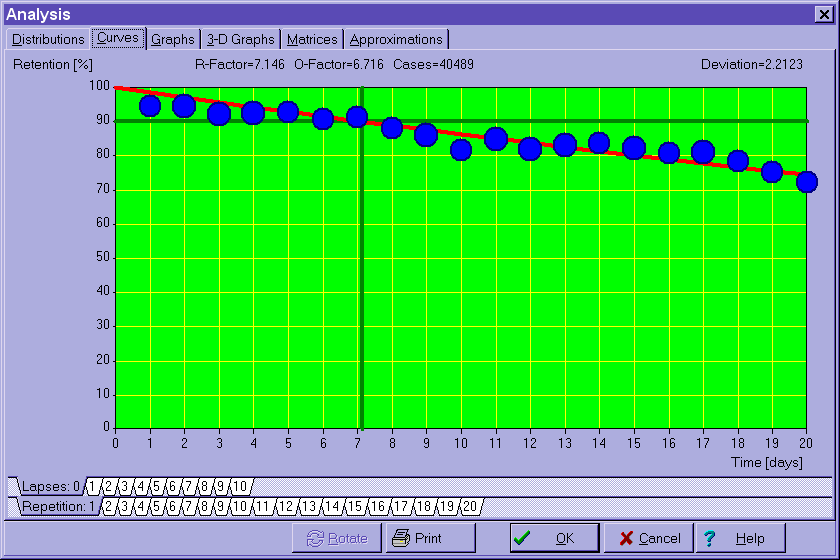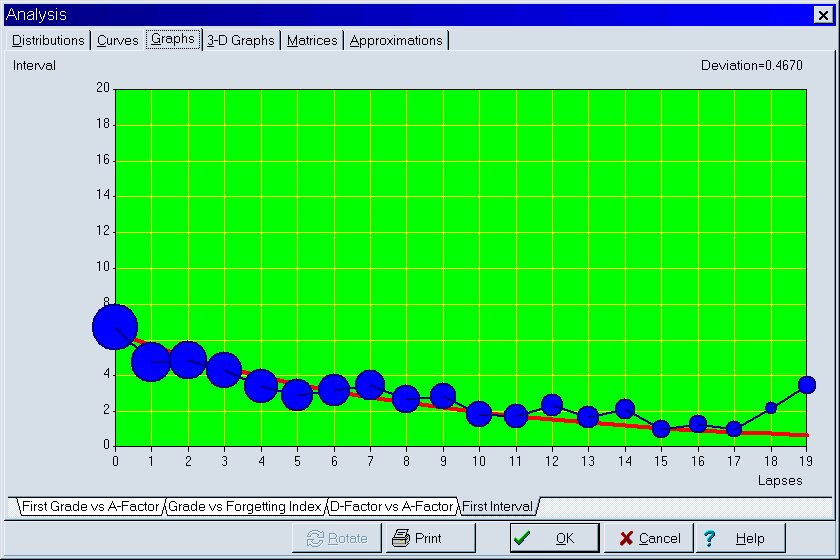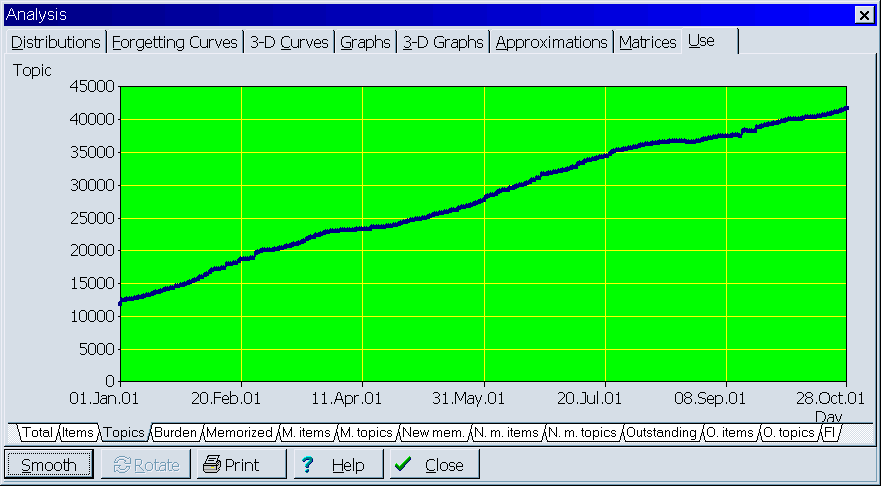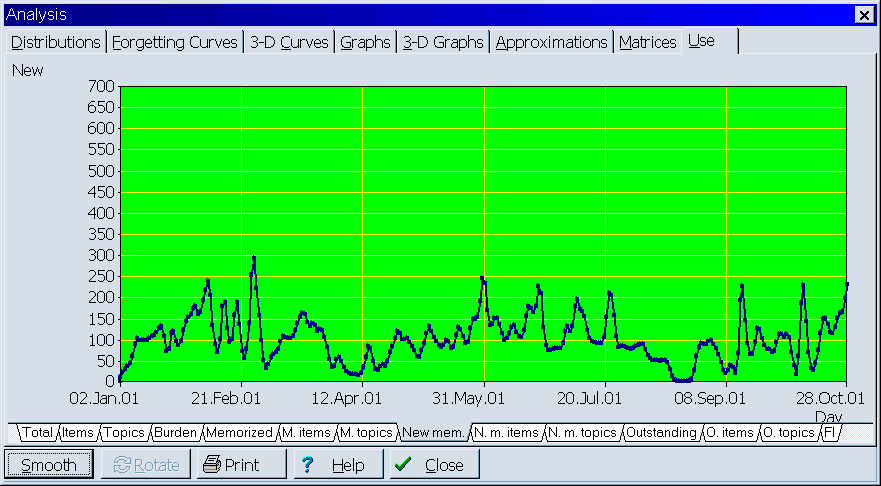## Analysis of the learning process

The tabbed dialog available from Tools : Statistics : Analysis in SuperMemo provides matrices and graphs that illustrate the current state of the learning process in the currently opened collection.

Some of these graph can be understood without understanding SuperMemo Algorithm; however, most of them require general understanding of how SuperMemo computes the optimum spacing of repetitions.
The following tabs are available in the Analysis dialog box:

Distributions

Interval distribution - distribution of inter-repetition intervals in a given collection. By changing the setting of the combo box at the bottom of the dialog, you can see interval distributions for topics, items or for all elements in the collection

A-Factor distribution - distribution of A-Factors in a given collection (note, that the distribution itself is not used in Algorithm SM-11, and merely results from it)

Repetitions distribution - distribution of the number of repetition in a given collection (only memorized elements are considered, i.e. there is no zero-repetitions category)

Lapses distribution - distribution of the number of times particular elements of the collection have been forgotten (only memorized elements are considered). Naturally, there are no lapses listed in this distribution if you select Topics only in the type combo box

Forgetting Curves - 400 forgetting curves are independently plotted in order to compute the RF matrix in Algorithm SM-11. These correspond to twenty repetition number categories multiplied by twenty A-Factor categories (note that for data representation convenience, the columns of the RF matrix for the first repetition are indexed by the number of memory lapses rather than A-Factor). By choosing a proper combination of tabs at the bottom of the graph, you can select a forgetting curve of interest. Horizontal axis represents time expressed as: (1) U-Factor, i.e. the ratio of subsequent inter-repetition intervals, or (2) days (only for the first repetition). Vertical axis represents knowledge retention as percentage:Blue circles in the picture represent data collected during repetitions. The greater the circle, the greater the number of repetitions recorded. The red curve corresponds with the best-fit forgetting curve obtained by exponential regression (note that for ill-structured material the forgetting curve is flattened, i.e. not exactly exponential). The horizontal green line corresponds with the requested forgetting index, while the vertical green line shows the moment in time in which the approximated forgetting curve intersects with the requested forgetting index line. This moment in time determines the value of the relevant R-Factor, and indirectly, the value of the optimum interval (for the first repetition, R-Factor corresponds with the optimum interval). The values of O-Factor and R-Factor are displayed at the top of the graph. They are followed by the number of repetition cases used to plot the graph. At the beginning of the learning process, there is no repetition history and no repetition data that could have been used to compute R-Factors. For that reason, the initial value of the RF matrix is taken from the model of a less-than-average student (the model of average student is not used because the convergence from poorer student parameters upwards is faster than the convergence in the opposite direction).

3-D Curves

These graphs show the forgetting curves in 3 dimensions. Each graph corresponds with a different A-Factor (from 1.2 to 6.9). The X axis represents time (as in Forgetting curves). The Y axis represents repetition category. The vertical Z axis represents retention in percent. For better visibility, you can rote 3-D forgetting curves with Rotate, or smooth them with Smooth. Note that smoothing proceeds along the Y axis. This makes it possible to align incompletely plotted forgetting curves from different repetition categories. However, once you click Rotate, Y axis smoothing will produce a different result. For best illustration of the forgetting process, smooth the graph before you rotate it.

Graphs

FI-G graph - G-FI graph correlates the expected forgetting index with the grade obtained at repetitions. You can imagine that the forgetting curve graph might use average grade instead of retention on its vertical axis. If you correlated this grade with the forgetting index (which is 100% minus retention), you arrive at the G-FI graph

G-AF graph - G-AF graph correlates the first grade obtained by an item with the ultimate estimation of its A-Factor value. At each repetition, the current element's old A-Factor estimation is removed from the graph and the new estimation is added. This graph is used by the SuperMemo Algorithm to quickly estimate the first value of A-Factor at the moment when all we know about an element is the first grade it has scored in its first repetition

DF-AF graph - DF-AF graph shows decay constants of power approximation of R-Factors along columns of the RF matrix. The horizontal axis represents A-Factor, while the vertical axis represents D-Factor (i.e. Decay Factor). D-Factor is a decay constant of power approximation of curves that can be inspected with the Approximations tab of the Analysis dialog box

First interval graph - the length of the first interval after the first repetition depends on the number of times a given item has been forgotten. Note that the first repetition here means the first repetition after forgetting (i.e. not the first repetition ever). In other words, a twice repeated item will have the repetition number equal to one after it has been forgotten (i.e. the repetition number will not equal three). The first interval graph shows exponential regression curve that approximates the length of the first interval for different numbers of memory lapses (including the zero-lapses category that corresponds with newly memorized items). In the graph below, blue circles correspond to data collected in the learning process (the greater the circle, the more repetitions have been recorded). In the presented case, newly memorized items are optimally repeated after seven days; however, items that have been forgotten 10 times (which is rare in SuperMemo) will require the interval of two days (due to logarithmic scaling, the size of the circle is not linearly proportional to the data sample and the number of repetition cases for lapses=0 is by far larger than for lapses=10 as can be seen in Distributions : Lapses distribution). The graph below includes data from over 90,000 repetitions3-D graphs that visually represent OF, RF and Cases matrices (see: Matrices)

Approximations

Twenty power approximation curves that show the decline of R-Factors along columns of the RF matrix. For each A-Factor, with increasing values of the repetition number, the value of R-Factor decreases (at least theoretically it should decrease). Power regression is used to illustrate the degree of this decline that is best reflected by the decay constant called here D-Factor. By choosing the A-Factor tab at the bottom of the graph, you can view a corresponding R-Factor approximation curve. The horizontal axis represents the repetition number, while the vertical axis represents R-Factor. The value of D-Factor is shown at the top of the graph. The blue polyline shows R-Factors as derived from repetition data. The red curve shows the fixed-point power approximation of R-Factor (fixed-point approach is used as for the repetition number equal two, R-Factor equals A-Factor). The green curve shows the fixed-point power approximation of R-Factor taken from the OF matrix. This is equivalent to substituting the D-Factor obtained by fixed-point power approximation of R-Factors with D-Factor obtained from DF-AF linear regression

Matrices

O-Factor matrix - matrix of optimal factors indexed by the repetition number and A-Factor (only for the first repetition, A-Factor is replaced with memory lapses)

R-Factor matrix - matrix of retention factors

Cases matrix - matrix of repetition cases used to compute the corresponding entries of the RF matrix (double click an entry to view the relevant forgetting curve). This matrix can be edited manually

Optimal intervals - matrix of optimum intervals derived from the OF matrix

D-Factor vector - vector of D-Factor values for different A-Factor values (also repetition cases used in computing particular D-Factors)

Use

Graphs of changes in the learning process statistics in time. These graph record daily changes to statistics such as the number of memorized items, measured forgetting index or the number of outstanding items for a given day. The first non-zero value of the day is recorded. If that value increases afterwards, that increase is ignored. If you have a break in learning, the last recorded non-zero value will propagate through the break period. Here are the statistics recorded daily by SuperMemo and displayed on the Use tab:

1. Total - total number of elements on a given day (i.e. topics + items + tasks)
2. Items - number of items in the collection
3. Topics - number of topics and tasks in the collection (see an exemplary graph below)
4. Burden - current Burden parameter record. Burden estimates the number of items scheduled for repetition daily on the assumption of no delays in the learning process. Burden is the sum of interval reciprocals
5. Memorized - number of elements in the learning process
6. Memorized items - number of items scheduled for repetition
7. Memorized topics - number of topics (and tasks) in incremental reading
8. New memorized elements - new elements memorized on a given day (see an exemplary smoothed graph below)
9. New memorized items - new items introduced into the learning process
10. New memorized topics - new topics introduced to incremental reading
11. Outstanding - number of outstanding elements scheduled for a given day
12. Outstanding items - number of items scheduled for repetition
13. Outstanding topics - number of topics scheduled for review
14. FI - measured forgetting index(Steven Trezise, USA, Apr 20, 1999)
Question:
In my collection, I have items for which I have done between 1 and 8 repetitions. However, when I look at the Cases matrix, there are no entries beyond repetition 3
The algorithm used by SuperMemo updates all optimization matrices using repetition categories, not the actual repetition number (you can view the optimization matrices with Tools : Statistics : Analysis : Matrices). A repetition category is an expected number of repetitions needed to reach the currently used interval. Once the matrices change, the estimation of repetition category may change too. If, for example, you score well in repetitions and your intervals become longer, it will take fewer repetitions to get to a given interval. In such a case, you might be at 8-th repetition while your repetition category will be 3. All matrices such as OF matrix, RF matrix, etc. will be updated in the third row (not in the 8-th row)

(Zoran Maximovic, Serbia, Sep 25, 2000)
Question:
In approximation graphs in Tools : Statistics : Analysis, some of the curves "jump out" of the graph area. What is wrong?